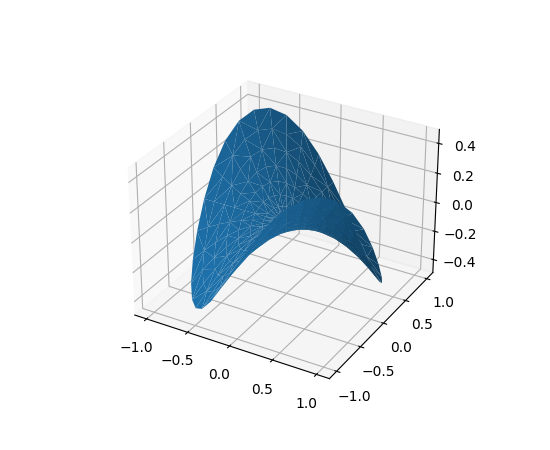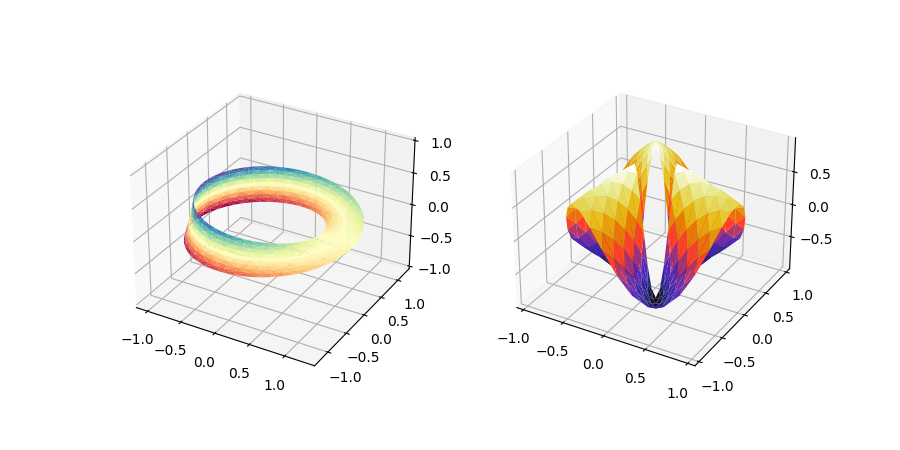# The mplot3d Toolkit¶

Generating 3D plots using the mplot3d toolkit.

## Getting started¶

3D Axes (of class Axes3D) are created by passing the projection="3d" keyword argument to Figure.add_subplot:

import matplotlib.pyplot as plt
fig = plt.figure()


Changed in version 1.0.0: Prior to Matplotlib 1.0.0, Axes3D needed to be directly instantiated with from mpl_toolkits.mplot3d import Axes3D; ax = Axes3D(fig).

Changed in version 3.2.0: Prior to Matplotlib 3.2.0, it was necessary to explicitly import the mpl_toolkits.mplot3d module to make the '3d' projection to Figure.add_subplot.

### Line plots¶

Axes3D.plot(self, xs, ys, *args, zdir='z', **kwargs)[source]

Plot 2D or 3D data.

Parameters: xs1D array-likex coordinates of vertices. ys1D array-likey coordinates of vertices. zsfloat or 1D array-likez coordinates of vertices; either one for all points or one for each point. zdir{'x', 'y', 'z'}, default: 'z'When plotting 2D data, the direction to use as z ('x', 'y' or 'z'). **kwargsOther arguments are forwarded to matplotlib.axes.Axes.plot.

### Scatter plots¶

Axes3D.scatter(self, xs, ys, zs=0, zdir='z', s=20, c=None, depthshade=True, *args, **kwargs)[source]

Create a scatter plot.

Parameters: xs, ysarray-likeThe data positions. zsfloat or array-like, default: 0The z-positions. Either an array of the same length as xs and ys or a single value to place all points in the same plane. zdir{'x', 'y', 'z', '-x', '-y', '-z'}, default: 'z'The axis direction for the zs. This is useful when plotting 2D data on a 3D Axes. The data must be passed as xs, ys. Setting zdir to 'y' then plots the data to the x-z-plane. See also Plot 2D data on 3D plot. sfloat or array-like, default: 20The marker size in points**2. Either an array of the same length as xs and ys or a single value to make all markers the same size. ccolor, sequence, or sequence of colors, optionalThe marker color. Possible values: A single color format string. A sequence of colors of length n. A sequence of n numbers to be mapped to colors using cmap and norm. A 2-D array in which the rows are RGB or RGBA. For more details see the c argument of scatter. depthshadebool, default: TrueWhether to shade the scatter markers to give the appearance of depth. Each call to scatter() will perform its depthshading independently. **kwargsAll other arguments are passed on to scatter. pathsPathCollection

### Wireframe plots¶

Axes3D.plot_wireframe(self, X, Y, Z, *args, **kwargs)[source]

Plot a 3D wireframe.

Note

The rcount and ccount kwargs, which both default to 50, determine the maximum number of samples used in each direction. If the input data is larger, it will be downsampled (by slicing) to these numbers of points.

Parameters: X, Y, Z2d arraysData values. rcount, ccountintMaximum number of samples used in each direction. If the input data is larger, it will be downsampled (by slicing) to these numbers of points. Setting a count to zero causes the data to be not sampled in the corresponding direction, producing a 3D line plot rather than a wireframe plot. Defaults to 50. New in version 2.0. rstride, cstrideintDownsampling stride in each direction. These arguments are mutually exclusive with rcount and ccount. If only one of rstride or cstride is set, the other defaults to 1. Setting a stride to zero causes the data to be not sampled in the corresponding direction, producing a 3D line plot rather than a wireframe plot. 'classic' mode uses a default of rstride = cstride = 1 instead of the new default of rcount = ccount = 50. **kwargsOther arguments are forwarded to Line3DCollection.

### Surface plots¶

Axes3D.plot_surface(self, X, Y, Z, *args, norm=None, vmin=None, vmax=None, lightsource=None, **kwargs)[source]

Create a surface plot.

By default it will be colored in shades of a solid color, but it also supports color mapping by supplying the cmap argument.

Note

The rcount and ccount kwargs, which both default to 50, determine the maximum number of samples used in each direction. If the input data is larger, it will be downsampled (by slicing) to these numbers of points.

Note

To maximize rendering speed consider setting rstride and cstride to divisors of the number of rows minus 1 and columns minus 1 respectively. For example, given 51 rows rstride can be any of the divisors of 50.

Similarly, a setting of rstride and cstride equal to 1 (or rcount and ccount equal the number of rows and columns) can use the optimized path.

Parameters: X, Y, Z2d arraysData values. rcount, ccountintMaximum number of samples used in each direction. If the input data is larger, it will be downsampled (by slicing) to these numbers of points. Defaults to 50. New in version 2.0. rstride, cstrideintDownsampling stride in each direction. These arguments are mutually exclusive with rcount and ccount. If only one of rstride or cstride is set, the other defaults to 10. 'classic' mode uses a default of rstride = cstride = 10 instead of the new default of rcount = ccount = 50. colorcolor-likeColor of the surface patches. cmapColormapColormap of the surface patches. facecolorsarray-like of colors.Colors of each individual patch. normNormalizeNormalization for the colormap. vmin, vmaxfloatBounds for the normalization. shadebool, default: TrueWhether to shade the facecolors. Shading is always disabled when cmap is specified. lightsourceLightSourceThe lightsource to use when shade is True. **kwargsOther arguments are forwarded to Poly3DCollection.

### Tri-Surface plots¶

Axes3D.plot_trisurf(self, *args, color=None, norm=None, vmin=None, vmax=None, lightsource=None, **kwargs)[source]

Plot a triangulated surface.

The (optional) triangulation can be specified in one of two ways; either:

plot_trisurf(triangulation, ...)


where triangulation is a Triangulation object, or:

plot_trisurf(X, Y, ...)
plot_trisurf(X, Y, triangles, ...)
plot_trisurf(X, Y, triangles=triangles, ...)


in which case a Triangulation object will be created. See Triangulation for a explanation of these possibilities.

The remaining arguments are:

plot_trisurf(..., Z)


where Z is the array of values to contour, one per point in the triangulation.

Parameters: X, Y, Zarray-likeData values as 1D arrays. colorColor of the surface patches. cmapA colormap for the surface patches. normNormalizeAn instance of Normalize to map values to colors. vmin, vmaxfloat, default: NoneMinimum and maximum value to map. shadebool, default: TrueWhether to shade the facecolors. Shading is always disabled when cmap is specified. lightsourceLightSourceThe lightsource to use when shade is True. **kwargsAll other arguments are passed on to Poly3DCollection

ExamplesNew in version 1.2.0.

### Contour plots¶

Axes3D.contour(self, X, Y, Z, *args, extend3d=False, stride=5, zdir='z', offset=None, **kwargs)[source]

Create a 3D contour plot.

Parameters: X, Y, Zarray-likeInput data. extend3dbool, default: FalseWhether to extend contour in 3D. strideintStep size for extending contour. zdir{'x', 'y', 'z'}, default: 'z'The direction to use. offsetfloat, optionalIf specified, plot a projection of the contour lines at this position in a plane normal to zdir. *args, **kwargsOther arguments are forwarded to matplotlib.axes.Axes.contour. matplotlib.contour.QuadContourSet

### Filled contour plots¶

Axes3D.contourf(self, X, Y, Z, *args, zdir='z', offset=None, **kwargs)[source]

Create a 3D filled contour plot.

Parameters: X, Y, Zarray-likeInput data. zdir{'x', 'y', 'z'}, default: 'z'The direction to use. offsetfloat, optionalIf specified, plot a projection of the contour lines at this position in a plane normal to zdir. *args, **kwargsOther arguments are forwarded to matplotlib.axes.Axes.contourf. matplotlib.contour.QuadContourSet

Notes

New in version 1.1.0: The zdir and offset parameters.

New in version 1.1.0: The feature demoed in the second contourf3d example was enabled as a result of a bugfix for version 1.1.0.

### Polygon plots¶

Axes3D.add_collection3d(self, col, zs=0, zdir='z')[source]

Add a 3D collection object to the plot.

2D collection types are converted to a 3D version by modifying the object and adding z coordinate information.

Supported are:
• PolyCollection
• LineCollection
• PatchCollection

### Bar plots¶

Axes3D.bar(self, left, height, zs=0, zdir='z', *args, **kwargs)[source]

Parameters: left1D array-likeThe x coordinates of the left sides of the bars. height1D array-likeThe height of the bars. zsfloat or 1D array-likeZ coordinate of bars; if a single value is specified, it will be used for all bars. zdir{'x', 'y', 'z'}, default: 'z'When plotting 2D data, the direction to use as z ('x', 'y' or 'z'). **kwargsOther arguments are forwarded to matplotlib.axes.Axes.bar. mpl_toolkits.mplot3d.art3d.Patch3DCollection

### Quiver¶

Axes3D.quiver(X, Y, Z, U, V, W, /, length=1, arrow_length_ratio=0.3, pivot='tail', normalize=False, **kwargs)[source]

Plot a 3D field of arrows.

The arguments could be array-like or scalars, so long as they they can be broadcast together. The arguments can also be masked arrays. If an element in any of argument is masked, then that corresponding quiver element will not be plotted.

Parameters: X, Y, Zarray-likeThe x, y and z coordinates of the arrow locations (default is tail of arrow; see pivot kwarg). U, V, Warray-likeThe x, y and z components of the arrow vectors. lengthfloat, default: 1The length of each quiver. arrow_length_ratiofloat, default: 0.3The ratio of the arrow head with respect to the quiver. pivot{'tail', 'middle', 'tip'}, default: 'tail'The part of the arrow that is at the grid point; the arrow rotates about this point, hence the name pivot. normalizebool, default: FalseWhether all arrows are normalized to have the same length, or keep the lengths defined by u, v, and w. **kwargsAny additional keyword arguments are delegated to LineCollection

### Text¶

Axes3D.text(self, x, y, z, s, zdir=None, **kwargs)[source]

Add text to the plot. kwargs will be passed on to Axes.text, except for the zdir keyword, which sets the direction to be used as the z direction.

### Subplotting¶

Having multiple 3D plots in a single figure is the same as it is for 2D plots. Also, you can have both 2D and 3D plots in the same figure.

New in version 1.0.0: Subplotting 3D plots was added in v1.0.0. Earlier version can not do this.

Keywords: matplotlib code example, codex, python plot, pyplot Gallery generated by Sphinx-Gallery